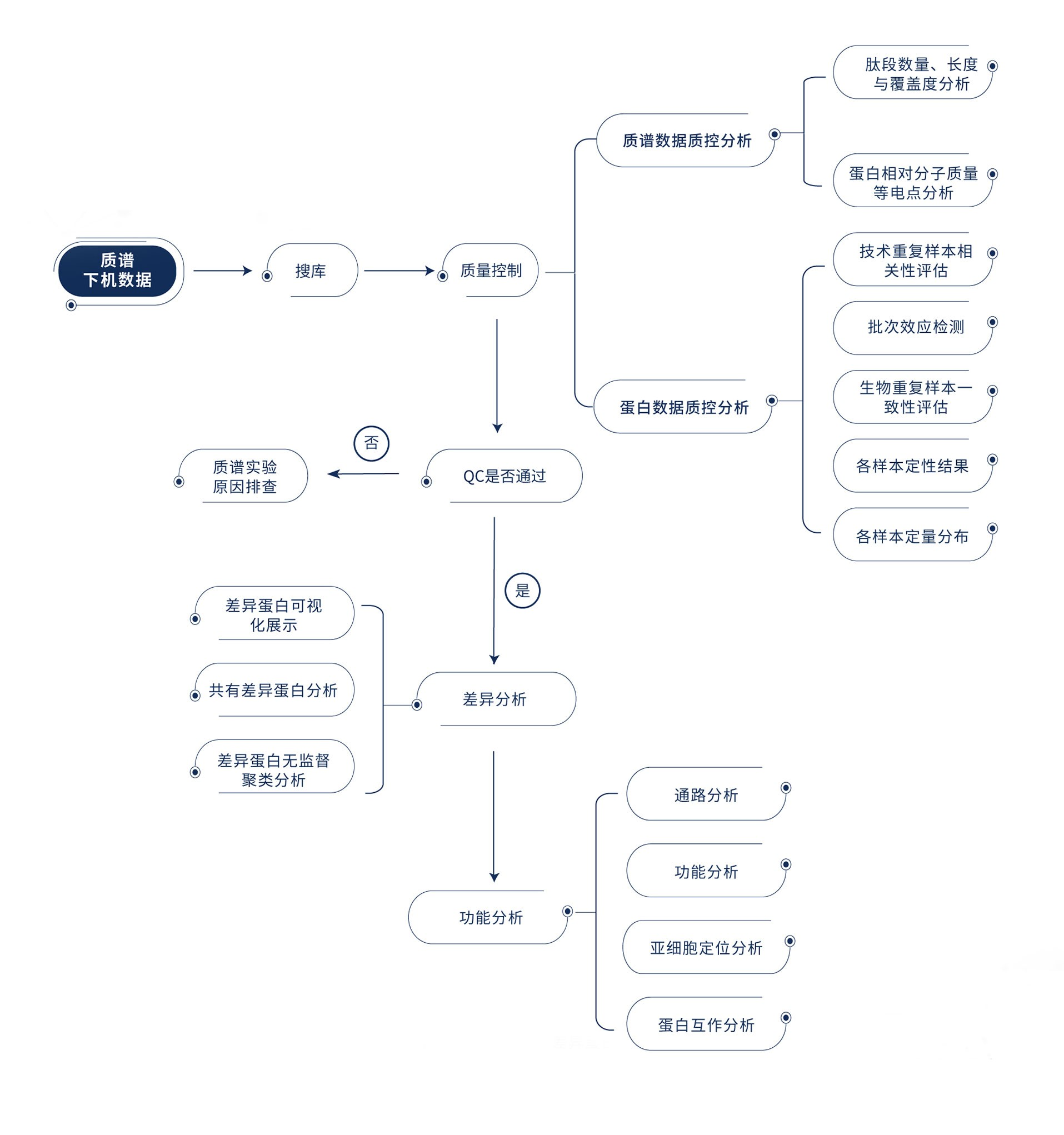The content of the standard bioinformatics analysis of Westlake Omics is as follows.

 Analysis content Explain Analysis of quality control Bar Plot, Density Plot, Correlation Heatmap, Correlation Scatter Plot,QC sample quantitative correlation analysis, Batch effect view Quantitative analysis Heatmap Differential analysis Volcano map，PCA； Function/pathway analysis GO、KEGG、PPIThe content of the standard bioinformatics analysis of Westlake Omics is as follows.

 Analysis content Explain Analysis of quality control Bar Plot, Density Plot, Correlation Heatmap, Correlation Scatter Plot,QC sample quantitative correlation analysis, Batch effect view Quantitative analysis Heatmap Differential analysis Volcano map，PCA； Function/pathway analysis GO、KEGG、PPI

Online enquiries